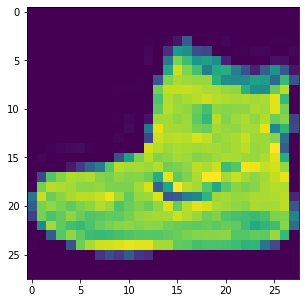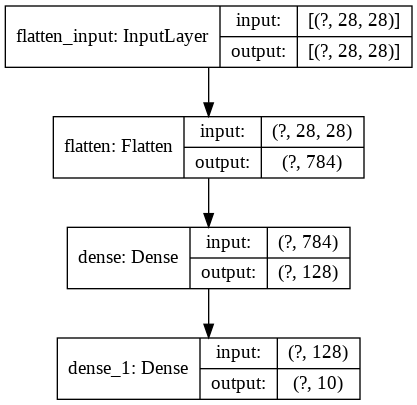fashion_mnist

# TensorFlow Tutorial Using Fashion MNIST (A Beginners Guide)¶Figure 1 Fashion-MNIST samples (by Zalando, MIT License).

## Introduction¶

Machine learning (ML)/Deep Learning(DL)/Neural Network (NN) tools have recently made a huge splash with applications in data analysis, image classification, and data generation. Although ML methods have existed for decades, recent advancements in hardware have generated systems powerful enough to run these algorithms.

The typical "hello world" example for ML is a classifier trained over the MNIST(Modified National Institute of Standards and Technology database) dataset; a dataset of the handwritten digits 0-9. This dataset is getting a little stale and is no longer impressive due to both its seeming simplicity and to the plethora of existing tutorials on the topic. Here we will use a newer dataset to perform our ML "hello world", the Fashion MNIST dataset!

The Zeroth Step of ML (that should be completed before ever putting a hand to mouse, or finger to key) is understanding the format and sizes of your data. This step is often referred to as feature engineering. Feature engineering, typically, includes selecting and preprocessing the particular aspects of training data to give to your algorithm. You and I will start a good habit of examining the data and its format to make decisions concerning the appropriate size and format for our NN.

The Fashion MNIST dataset is comprised of 70,000 grayscale images of articles of clothing. The greyscale values for a pixel range from 0-255 (black to white). Each low-resolution image is 28x28 pixels and is of exactly one clothing item. Alongside each image is a label that places the article within a category; these categories are shown in Figure 2 with an example image belonging to the class.Figure 2 class numbers are shown next to image labels

## TL;DR¶

Open this tutorial on Google colab:And, walk through the tutorial yourself.

Also check out DataCamp if you want to learn Data Science and Machine Learning Online for FREE like I did.

## Code walk-through¶

To complete this tutorial we will to install a few packages; pip is a fast and easy way to install packages and their dependencies. For Machine Learning projects we use a library called TensorFlow, it handle all the complicated maths for you so you do not need to worry much on the maths side of things. For visualization we using matplotlib & nnv and for numerical-computations we using numpy.

In [ ]:


In [ ]:
!pip install tensorflow
!pip install matplotlib
!pip install numpy
!pip install nnv

Requirement already satisfied: tensorflow in /usr/local/lib/python3.6/dist-packages (2.3.0)
Requirement already satisfied: h5py<2.11.0,>=2.10.0 in /usr/local/lib/python3.6/dist-packages (from tensorflow) (2.10.0)
Requirement already satisfied: opt-einsum>=2.3.2 in /usr/local/lib/python3.6/dist-packages (from tensorflow) (3.3.0)
Requirement already satisfied: scipy==1.4.1 in /usr/local/lib/python3.6/dist-packages (from tensorflow) (1.4.1)
Requirement already satisfied: termcolor>=1.1.0 in /usr/local/lib/python3.6/dist-packages (from tensorflow) (1.1.0)
Requirement already satisfied: tensorflow-estimator<2.4.0,>=2.3.0 in /usr/local/lib/python3.6/dist-packages (from tensorflow) (2.3.0)
Requirement already satisfied: protobuf>=3.9.2 in /usr/local/lib/python3.6/dist-packages (from tensorflow) (3.12.4)
Requirement already satisfied: keras-preprocessing<1.2,>=1.1.1 in /usr/local/lib/python3.6/dist-packages (from tensorflow) (1.1.2)
Requirement already satisfied: grpcio>=1.8.6 in /usr/local/lib/python3.6/dist-packages (from tensorflow) (1.32.0)
Requirement already satisfied: wrapt>=1.11.1 in /usr/local/lib/python3.6/dist-packages (from tensorflow) (1.12.1)
Requirement already satisfied: wheel>=0.26 in /usr/local/lib/python3.6/dist-packages (from tensorflow) (0.35.1)
Requirement already satisfied: tensorboard<3,>=2.3.0 in /usr/local/lib/python3.6/dist-packages (from tensorflow) (2.3.0)
Requirement already satisfied: absl-py>=0.7.0 in /usr/local/lib/python3.6/dist-packages (from tensorflow) (0.10.0)
Requirement already satisfied: numpy<1.19.0,>=1.16.0 in /usr/local/lib/python3.6/dist-packages (from tensorflow) (1.18.5)
Requirement already satisfied: astunparse==1.6.3 in /usr/local/lib/python3.6/dist-packages (from tensorflow) (1.6.3)
Requirement already satisfied: six>=1.12.0 in /usr/local/lib/python3.6/dist-packages (from tensorflow) (1.15.0)
Requirement already satisfied: gast==0.3.3 in /usr/local/lib/python3.6/dist-packages (from tensorflow) (0.3.3)
Requirement already satisfied: setuptools in /usr/local/lib/python3.6/dist-packages (from protobuf>=3.9.2->tensorflow) (50.3.0)
Requirement already satisfied: werkzeug>=0.11.15 in /usr/local/lib/python3.6/dist-packages (from tensorboard<3,>=2.3.0->tensorflow) (1.0.1)
Requirement already satisfied: markdown>=2.6.8 in /usr/local/lib/python3.6/dist-packages (from tensorboard<3,>=2.3.0->tensorflow) (3.2.2)
Requirement already satisfied: requests<3,>=2.21.0 in /usr/local/lib/python3.6/dist-packages (from tensorboard<3,>=2.3.0->tensorflow) (2.23.0)
Requirement already satisfied: tensorboard-plugin-wit>=1.6.0 in /usr/local/lib/python3.6/dist-packages (from tensorboard<3,>=2.3.0->tensorflow) (1.7.0)
Requirement already satisfied: importlib-metadata; python_version < "3.8" in /usr/local/lib/python3.6/dist-packages (from markdown>=2.6.8->tensorboard<3,>=2.3.0->tensorflow) (2.0.0)
Requirement already satisfied: rsa<5,>=3.1.4; python_version >= "3" in /usr/local/lib/python3.6/dist-packages (from google-auth<2,>=1.6.3->tensorboard<3,>=2.3.0->tensorflow) (4.6)
Requirement already satisfied: chardet<4,>=3.0.2 in /usr/local/lib/python3.6/dist-packages (from requests<3,>=2.21.0->tensorboard<3,>=2.3.0->tensorflow) (3.0.4)
Requirement already satisfied: urllib3!=1.25.0,!=1.25.1,<1.26,>=1.21.1 in /usr/local/lib/python3.6/dist-packages (from requests<3,>=2.21.0->tensorboard<3,>=2.3.0->tensorflow) (1.24.3)
Requirement already satisfied: certifi>=2017.4.17 in /usr/local/lib/python3.6/dist-packages (from requests<3,>=2.21.0->tensorboard<3,>=2.3.0->tensorflow) (2020.6.20)
Requirement already satisfied: idna<3,>=2.5 in /usr/local/lib/python3.6/dist-packages (from requests<3,>=2.21.0->tensorboard<3,>=2.3.0->tensorflow) (2.10)
Requirement already satisfied: zipp>=0.5 in /usr/local/lib/python3.6/dist-packages (from importlib-metadata; python_version < "3.8"->markdown>=2.6.8->tensorboard<3,>=2.3.0->tensorflow) (3.2.0)
Requirement already satisfied: pyasn1>=0.1.3 in /usr/local/lib/python3.6/dist-packages (from rsa<5,>=3.1.4; python_version >= "3"->google-auth<2,>=1.6.3->tensorboard<3,>=2.3.0->tensorflow) (0.4.8)
Requirement already satisfied: matplotlib in /usr/local/lib/python3.6/dist-packages (3.2.2)
Requirement already satisfied: pyparsing!=2.0.4,!=2.1.2,!=2.1.6,>=2.0.1 in /usr/local/lib/python3.6/dist-packages (from matplotlib) (2.4.7)
Requirement already satisfied: numpy>=1.11 in /usr/local/lib/python3.6/dist-packages (from matplotlib) (1.18.5)
Requirement already satisfied: cycler>=0.10 in /usr/local/lib/python3.6/dist-packages (from matplotlib) (0.10.0)
Requirement already satisfied: kiwisolver>=1.0.1 in /usr/local/lib/python3.6/dist-packages (from matplotlib) (1.2.0)
Requirement already satisfied: python-dateutil>=2.1 in /usr/local/lib/python3.6/dist-packages (from matplotlib) (2.8.1)
Requirement already satisfied: six in /usr/local/lib/python3.6/dist-packages (from cycler>=0.10->matplotlib) (1.15.0)
Requirement already satisfied: numpy in /usr/local/lib/python3.6/dist-packages (1.18.5)
Requirement already satisfied: nnv in /usr/local/lib/python3.6/dist-packages (0.0.4)

In [ ]:
import tensorflow as tf
from tensorflow import keras

import matplotlib.pyplot as plt
import numpy as np


## Import MNIST¶

Now we are ready to roll! First, we must admit that it takes a lot of data to train a NN, and 70,000 examples is an anemic dataset. So instead of doing a more traditional 70/20/10 or 80/10/10 percent split between training/validating/testing, we will do a simple 6:1 ratio of training:testing (note that this is not best practices, but when there is limited data it may be your only recourse).

We first load in the dataset from the Keras package:

In [ ]:
fashion_mnist = keras.datasets.fashion_mnist
(train_images, train_labels), (test_images, test_labels) = fashion_mnist.load_data()


The first line merely assigns the name fashion_mnist to a particular dataset located within Keras' dataset library. The second line defines four arrays containing the training and testing data, cleaved again into separate structures for images and labels, and then loads all of that data into our standup of Python. The training data arrays will be used to --you guessed it-- train the model, and the testing arrays will allow us to evaluate the performance of our model.

## Look at data¶

It's always nice to be able to show that we've actually done something; ever since kindergarten there has been no better way than with a picture! You'll note that we pip installed and imported MatPlotLib, a library for plots and graphs. Here we'll use it to visualize an example of the Fashion MNIST dataset.

In [ ]:
print(f"Train images dimensions: {train_images.shape}")
print(f"Test images dimensions: {test_images.shape}")

Train images dimensions: (60000, 28, 28)
Test images dimensions: (10000, 28, 28)


The first command basically generates a figure object that will be manipulated by commands 2 through 4. Command 2 specifies what it is that we shall be plotting: the first element from the train_images array. NOTE: Recall that python is an inclusive counting language, meaning that it numbers/indexes things starting from zero, not one! And the final command, "show()", tells Python to generate this figure in an external (from CMD) window.

Your window should contain a plot that looks similar to Figure 3. Also, be aware that after plt.show(), Python will not return you to a command line until the newly generated window (containing our super nice picture) is closed. Upon closing the window, you will be able to continue entering Python commands.

In [ ]:
plt.figure(figsize=(10,5))
plt.imshow(train_images)
plt.colormaps()
plt.show()## Preprocessing the dataset¶

The greyscale assigned to each pixel within an image has a value range of 0-255. We will want to flatten (smoosh… scale…) this range to 0-1. To achieve this flattening, we will exploit the data structure that our images are stored in, arrays. You see, each image is stored as a 2-dimensional array where each numerical value in the array is the greyscale code of particular pixel. Conveniently, if we divide an entire array by a scalar we generate a new array whose elements are the original elements divided by the scalar.

In [ ]:
train_images = train_images /255.
test_images = test_images / 255


## Model Generation¶

Every Neural Network is constructed from a series of connected layers that are full of connection nodes. Simple mathematical operations are undertaken at each node in each layer, yet through the volume of connections and operations, these ML models can perform impressive and complex tasks.

To read and understand more about the underlying maths, checkout some of the notes I made when I took the deeplearning.ai course here.

Our model will be constructed from 3 layers. The first layer – often referred to as the Input Layer – will intake an image and format the data structure in a method acceptable for the subsequent layers. In our case, this first layer will be a Flatten layer that intakes a multi-dimensional array and produces an array of a single dimension, this places all the pixel data on an equal depth during input. Both of the next layers will be simple fully connected layers, referred to as Dense layers, with 128 and 10 nodes respectively. These fully connected layers are the simplest layer in the sense of understanding, yet allow for the greatest number of layer-to-layer connections and relationships.

The final bit of hyper-technical knowledge you'll need to learn is that each layer can have its own particular mathematical operation. These activation functions determine the form and relationship between the information provided by the layer. The first dense layer will feature a Rectified Linear Unit (ReLU) Activation Function that outputs values between zero and 1; mathematically, the activation function behaves like f(x)=max(0,x). The final layer uses the softmax activation function. This function also produces values in the 0-1 range, BUT generates these values such that the sum of the outputs will be 1!

This makes the softmax a layer that is excellent at outputting probabilities.

I found this lecture video very insightful into why we need to flatten the array.

In [ ]:
model = keras.Sequential([
keras.layers.Flatten(input_shape=(28, 28)),
keras.layers.Dense(128, activation=tf.nn.relu),
keras.layers.Dense(10, activation=tf.nn.softmax)
])


Running the code below creates a file model_plot.png with a plot of the created model.

In [ ]:
keras.utils.plot_model(model, to_file='model.png', show_shapes=True)

Out[ ]:Let's visualise how the neural network feed fordward would look:

In [ ]:
from nnv import NNV
plt.rcParams["figure.figsize"] = 200,50

layersList = [
{"title":"Input\n(784 flatten)", "units": 784, "color": "Blue"},
{"title":"Hidden 1\n(relu: 128)", "units": 128},
{"title":"Output\n(softmax: 10)", "units": 10,"color": "Green"},
]